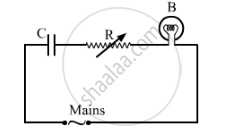# A Capacitor 'C', a Variable Resistor 'R' and a Bulb 'B' Are Connected in Series to the Ac Mains in Circuit - Physics

A capacitor 'C', a variable resistor 'R' and a bulb 'B' are connected in series to the ac mains in circuit as shown. The bulb glows with some brightness. How will the glow of the bulb change if (i) a dielectric slab is introduced between the plates of the capacitor, keeping resistance R to be the same; (ii) the resistance R is increased keeping the same capacitance?#### Solution

(i)As the dielectric slab is introduced between the plates of the capacitor, its capacitance will increase. Hence, the potential drop across the capacitor will decrease (V=Q/C). As a result, the potential drop across the bulb will increase (since both are connected in series). So, its brightness will increase.

(ii)As the resistance (R) is increased, the potential drop across the resistor will increase. As a result, the potential drop across the bulb will decrease (since both are connected in series).
So, its brightness will decrease.

Concept: Combination of Capacitors
Is there an error in this question or solution?